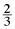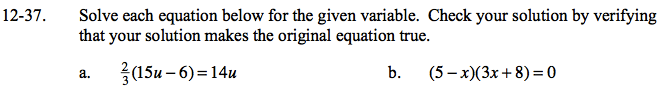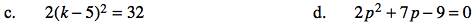### Home > GC > Chapter 12 > Lesson 12.1.4 > Problem12-37

12-37.
1. Solve each equation below for the given variable. Check your solution by verifying that your solution makes the original equation true. Homework Help ✎

1.(15u − 6) = 14u

2. (5 − x)(3x + 8) = 0

3. 2(k − 5)2 = 32

4. 2p2 + 7p − 9 = 0Distribute before simplifying.

10u − 4 = 14u
− 4 = 4u
u = − 1

Use the Zero Product Property.

$x=5, - \frac{ 8 }{3 }$Divide by 2 first and be sure to find two solutions.

k = 1 or 9

$\frac{ -7\pm \sqrt{ (7)^{2}-4(2)(-9) } }{4(2) } = \frac{-7 \pm \sqrt{121}}{8}$